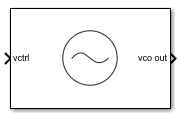# VCO

Model voltage controlled oscillator

• Library:
• Mixed-Signal Blockset / PLL / Building Blocks

•## Description

VCO or voltage controlled oscillator is a voltage to frequency converter. It produces an output signal whose frequency is controlled by the voltage at the input vctrl port. The frequency of the output signal, F is determined either by:

$F=\left({K}_{VCO}·{V}_{ctrl}\right)+{F}_{o}$

where:

• Kvco = voltage sensitivity (in Hz/V)

• Vctrl = control voltage (in V)

• Fo= free running frequency (in Hz)

or from linear interpolation using the mapping:

$F=\text{interp}\left({F}_{out}\left({V}_{cntl}\right)\right)$

where:

• Vctnl = vector of control voltages (in V)

• Fout= vector of corresponding output frequencies (in Hz)

## Ports

### Input

expand all

VCO control voltage used to generate output frequency of VCO. In a phase-locked loop (PLL) system, vctrl is the output of the Loop Filter that contains the phase error information.

Data Types: `double`

### Output

expand all

Output signal of VCO. In a PLL system, vco out is the output clock generated by the PLL. It is also fed back to the PFD block through a clock divider to complete the control loop.

Data Types: `double`

## Parameters

expand all

### Configuration

Define how VCO output frequency is specified:

• Select `Voltage sensitivity` to specify output frequency from Voltage sensitivity (Hz/V) and Free running frequency (Hz).

• Select ```Output frequency vs. control voltage``` to interpolate output frequency from Control voltage (V) vector versus Output frequency (Hz) vector.

#### Programmatic Use

• Use `set_param(gcb,'SpecifyUsing','Voltage sensitivity')` to set Specify using to `Voltage sensitivity`.

• Use `set_param(gcb,'SpecifyUsing', 'Output frequency vs. control voltage')` to set Specify using to ```Output frequency vs. control voltage```.

Measure of change in output frequency for input voltage change, specified as a positive real scalar with units in Hz/V. This parameter is also reported as VCO voltage sensitivity in the Loop Filter tab and is used to automatically calculate the filter component values of the loop filter.

#### Dependencies

To enable this parameter, select ```Voltage sensitivity``` in Specify using in the VCO tab.

#### Programmatic Use

• Use `get_param(gcb,'Kvco')` to view the current Voltage sensitivity (Hz/V) value.

• Use `set_param(gcb,'Kvco',value)` to set Voltage sensitivity (Hz/V) to a specific value.

Data Types: `double`

Frequency of the VCO without any control voltage input (`0` V), or the quiescent frequency, specified as a positive real scalar in Hz.

#### Dependencies

To enable this parameter, select ```Voltage sensitivity``` in Specify using in the VCO tab.

#### Programmatic Use

• Use `get_param(gcb,'Fo')` to view current Free running frequency (Hz) value.

• Use `set_param(gcb,'Fo',value)` to set Free running frequency (Hz) to a specific value.

Data Types: `double`

Control voltage values of the VCO, specified as a real valued vector in volts.

#### Dependencies

To enable this parameter, select ```Output frequency vs. control voltage``` in Specify using in the VCO tab.

#### Programmatic Use

• Use `get_param(gcb,'ControlVoltage')` to view current Control voltage (V) value.

• Use `set_param(gcb,'ControlVoltage',value)` to set Control voltage (V) to a specific value.

Data Types: `double`

Output frequency of the values of the VCO, corresponding to the Control voltage (V) vector, specified in Hz.

#### Dependencies

To enable this parameter, select ```Output frequency vs. control voltage``` in Specify using in the VCO tab.

#### Programmatic Use

• Use `get_param(gcb,'OutputFrequency')` to view current Output frequency (Hz) value.

• Use `set_param(gcb,'OutputFrequency',value)` to set Output frequency (Hz) to a specific value.

Data Types: `double`

Maximum amplitude of the VCO output voltage, specified as a positive real scalar.

#### Programmatic Use

• Use `get_param(gcb,'Amplitude')` to view current Output amplitude (V) value.

• Use `set_param(gcb,'Amplitude',value)` to set Output amplitude (V) to a specific value.

Data Types: `double`

Select to enable increased buffer size during simulation. This increases the buffer size of the Variable Pulse Delay block inside the VCO block. By default, this option is deselected.

Number of samples of the input buffering available during simulation, specified as a positive integer scalar. This sets the buffer size of the Variable Pulse Delay block inside the VCO block.

Selecting different simulation solver or sampling strategies can change the number of input samples needed to produce an accurate output sample. Set the Buffer size to a large enough value so that the input buffer contains all the input samples required.

#### Dependencies

This parameter is only available when Enable increased buffer size option is selected in the Configuration tab.

#### Programmatic Use

• Use `get_param(gcb,'NBuffer')` to view the current value of Buffer size.

• Use `set_param(gcb,'NBuffer',value)` to set Buffer size to a specific value.

Data Types: `double`

### Impairments

Select to introduce phase noise as a function of frequency to the VCO. By default, this option is selected.

The frequency offsets of phase noise from the carrier frequency specified as a real valued vector in Hz.

#### Dependencies

To enable this parameter, select Add phase noise in the Impairments tab.

#### Programmatic Use

• Use `get_param(gcb,'Foffset')` to view current Phase noise frequency offset (Hz) metric.

• Use `set_param(gcb,'Foffset',value)` to set Phase noise frequency offset (Hz) to a specific metric.

Data Types: `double`

The phase noise power in a 1 Hz bandwidth centered at the specified frequency offsets relative to the carrier specified as a real valued vector in dBc/Hz. The elements of Phase noise level corresponds to relative elements in the Phase noise frequency offset.

#### Dependencies

To enable this parameter, select Add phase noise in the Impairments tab.

#### Programmatic Use

• Use `get_param(gcb,'PhaseNoise')` to view current Phase noise level (dBc/Hz) metric.

• Use `set_param(gcb,'PhaseNoise',value)` to set Phase noise level (dBc/Hz) to a specific metric.

Data Types: `double`